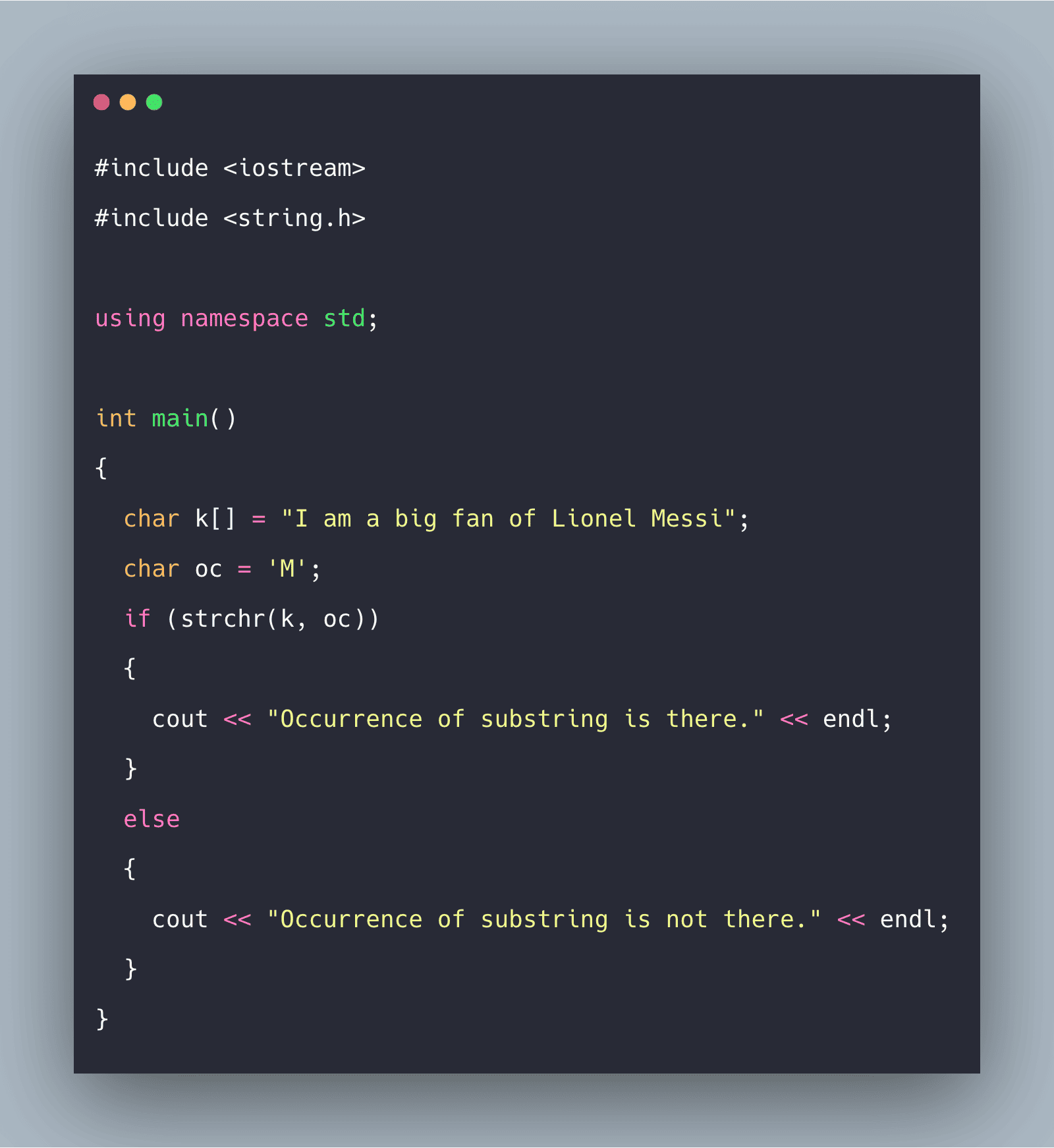# C++ strchr() Function: Complete Guide

0
3The strchr() function finds the first occurrence of a character static_cast<char>(ch) in the byte string pointed by str. If the occurrence is found a pointer to the location of that particular character in the string is returned.

## C++ strchr() Function

C++ strchr() is a built-in function that is used for string handling, and it is defined under the string.h header file. The strchr() function is used for finding the first occurrence of a character in a particular string. The terminating null character is considered to be the part of the string and can be found if searching for ‘\0’.

### Syntax

```char *strchr(const char *str_var, int oc);
```

### Parameters

The strchr() takes two parameters.

1. The first one is the str_var which is the main string.
2. The second one is oc which is the character of finding the occurrence in the main string. Initially oc variable is of the char data type. It mainly checks the occurrence of oc in the string which is pointed by str_var.

### Return Value

It returns a pointer to the location of the character’s occurrence in the main string.

### Examples of strchr() function in C++

#### Example 1: Write a program to show the working of strchr() in C++

```#include <iostream>
#include <string.h>

using namespace std;

int main()
{
char k[] = "I am a big fan of Lionel Messi";
char oc = 'M';
if (strchr(k, oc))
{
cout << "Occurrence of substring is there." << endl;
}
else
{
cout << "Occurrence of substring is not there." << endl;
}
}```

#### Output

```Occurrence of substring is there.
```

#### Example 2: Take a string and character, make sure that the character is not present in the string. Show the output using strchr() function.

```#include <iostream>
#include <string.h>

using namespace std;

int main()
{
char k[] = "I am a big fan of Lionel Messi";
char oc = 'K';
if (strchr(k, oc))
{
cout << "Occurrence of substring is there." << endl;
}
else
{
cout << "Occurrence of substring is not there." << endl;
}
}```

#### Output

```Occurrence of substring is not there.
```

## Conclusion

C++ strchr() function takes two arguments: str and ch. The function searches for the character ch in the string pointed to by str. The function is defined in <cstring> header file.

This site uses Akismet to reduce spam. Learn how your comment data is processed.